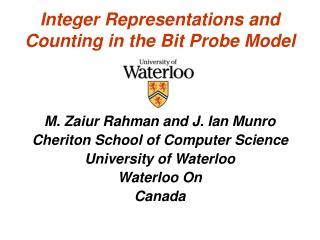# Integer Representations and Counting in the Bit Probe Model - PowerPoint PPT PresentationDownload PresentationInteger Representations and Counting in the Bit Probe Model

Download Presentation## Integer Representations and Counting in the Bit Probe Model

- - - - - - - - - - - - - - - - - - - - - - - - - - - E N D - - - - - - - - - - - - - - - - - - - - - - - - - - -
##### Presentation Transcript

1. Integer Representations and Counting in the Bit Probe Model M. Zaiur Rahman and J. Ian Munro Cheriton School of Computer Science University of Waterloo Waterloo On Canada

2. Integer Representations • Standard: n bits representing {0..2n} • # bits … optimal • Increment: • Θ(n) bit inspections/changes worst case • O(1) amortized, but not if we include decrement

3. Redundant Binary Numbers • Use “digits” 0,1,2 but representation is in binary, 2 is a delayed carry e.g. 01220 = 10100 • Space 2n bits (or reduce to about (lg 3) n) • Increment O(1), as we de-amortize the scan to release the rightmost carry • Decrement is tricky, easiest to use digit “-1” • Note the extra lg n bits for the scan pointer

4. Gray Codes.. Due to Gray..of course Binary Reflected Gray Code (BRGC) • Of dimension n (i.e. n bits, numbers [0..2n] • A sequence of 2n strings each of length n G(n) = 0.G(n-1), 1.G(n-1)R (reversal is on thesequence order, not the individual codes)

5. Increment on a Gray Code • Even parity: flip rightmost 1 bit

6. Increment on a Gray Code • Even parity: flip rightmost bit 00110000 → 00110001

7. Increment on a Gray Code • Even parity: flip rightmost bit 00110000 → 00110001 • Odd parity: flip the bit to left of rightmost 1 01110000 → 01010000

8. Increment on a Gray Code • Even parity: flip rightmost bit 00110000 → 00110001 • Odd parity: flip the bit to left of rightmost 1 01110000 → 01010000 • Costs:1 bit changed, n inspections (worst case) • Or: add parity bit, O(1) amortized insert, 2 bits changed • Integrate parity bit with code (no extra bit [Lucal])

9. Our First Result: Lower Bound Theorem: Any representation of [0..2n] using exactly n bits requires Ω(√n) bit inspections in the worst case. Proof: Model, apply Sunflower Lemma and manipulate

10. Sunflower Lemma [Ёrdos & Rado] • Sunflower with p petals • Sets S1, …, Sp so SiSj is same for all i,j Lemma: S1, …, Sp is a system of sets each of size at most q. If m>(p-1)q+1q!, then it contains a subcollection with p petals Use the lemma with m as small as possible

11. Back to Algorithms • We want a scheme that balances (as well as possible) • Bit changes • Bit inspections • Extra bits Lower bound says no extra bits  lots of inspections

12. The increment in BRGC • Key issue … that rightmost 1 Lemma: On increment or decrement, the position of rightmost 1 in any segment of the leftmost bits changes only when that bit is flipped, except for the case of leftmost bit going 1→0 on increment (or 0 → 1 on decrement)

13. De-amortizing • The lemma lets us de-amortize: as with redundant binary representation. With some details: Theorem: There is a representation using n + lg n + 3 bits (a pointer is in there), requiring 2 lg n +4 bit inspections and 4 bit changes for increment or decrement.

14. Moving Along • Complicating issue:Mixing increments and decrements forces us to add flags • (for {inc, dec} and {done yet , not done} • Idea: use the lower order lg n bits to give the pointer into the leftmost n - lg n bits • This would seem to require a table (or model issue) to translate

15. But • Permute the code of the leading n – lg n bits and use trailing bits directly to walk through them in “code order” • Indeed, we don’t need n – lg n to be a power of 2 Theorem: There is a representation using n + 3 bits, requiring lg n + 6 bit inspections and 7 bit changes for increment or decrement.

16. Addition and Subtraction • Adding or subtracting and m digit (standard notation) integer to update an n digit integer (n > m): • n + O(lg2n) bits, • O(m + lg n) inspections/changes

17. Open Issues • Improve the lower bound to n bits inspected in no extra bits • Lower bound trading off bits changed with space and bits inspected • Tweaking upper bounds